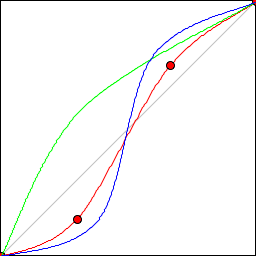# CurvesFilter

This filter applies a transfer curve to each channel of an image. The curve is defined as a series of x,y points to which a spline is fitted. For example, here are a set of red, green and blue curves:RGB Curves

...and here is the result of applying the filter:CurvesFilter

The diagram shows the four points used to define the red curve. There's no limit on the number of points used to define a curve. If you want to apply the same curve to each channel, you need only define a single curve.